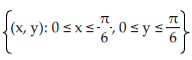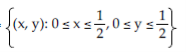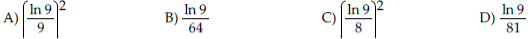/
/
/
21) f(x, y) = 6x + 8y; R = {(x,
Not my Question
Flag Content

# Question : 21) f(x, y) = 6x + 8y; R = {(x, : 1757211

21) f(x, y) = 6x + 8y; R = {(x, y): 0 x 4, 0 y 6}

A) 15 B) 36 C) 16 D) 12

22) f(x, y) = 10x + 3y over the triangle with vertices (0, 0), (9, 0), and (0, 2).

A) 32 B) 30 C) 31 D) 92/3

23) f(x, y) = 5x + 7y over the region bounded by the coordinate axes and the lines x + y = 5 and x + y = 10.

A) 140/3 B) 70/3 C) 35 D) 35/2

24) f(x, y) = sin 3(x + y); R =A) 6/π2 B) 4π2 C) π2 D) 8/π2

25) f(x, y) = e2x; R =A) e - 1/2 B) e - 1 C) 2e - 1/4 D) 2e - 1

26) f(x, y) = 1/xy ; R = {(x, y): 1 x 9, 1 y 9}27) f(x, y) = 1/ (xy)2; R = {(x, y): 1 x 6, 1 y 6}

A) 1/6 B) ln 6/6 C) 1/36 D) ln 6/36

28) f(x, y) = 1/xy over the region bounded by y = 1/x, y = 2/x , x = 4, and x = 6.

A) ln 2 B) 3/2 ln 2 C) 24 ln 2 D) 2/3 ln 2

29) f(x, y) = ex2 over the region bounded by y = 4x, y = 9x , x = 0, and x = 1.

A) e - 1 B) e - 1/4 C) 2e - 1/16 D) 2e - 1

30) The northern third of Indiana is a rectangle measuring 96 miles by 132 miles. Thus, let D = [0, 96] × [0, 132]. Assuming that the total annual snowfall (in inches), S(x,y), at (x,y) D is given by the function S(x,y) = 60e-0.001(2x + y) with (x,y) D, find the average snowfall on D.

A) 52.44 inches B) 51.78 inches C) 52.06 inches D) 51.14 inches

## Solution 5 (1 Ratings )

Solved
Mathematics 3 Years Ago 63 Views